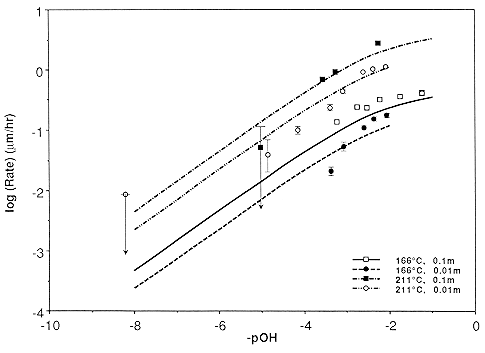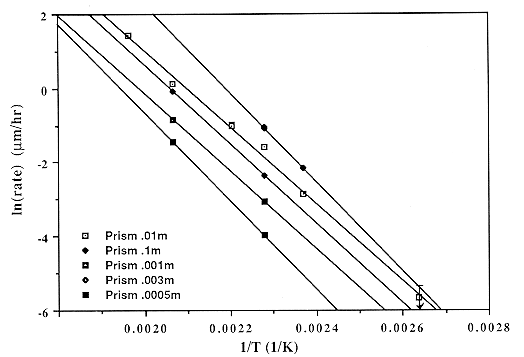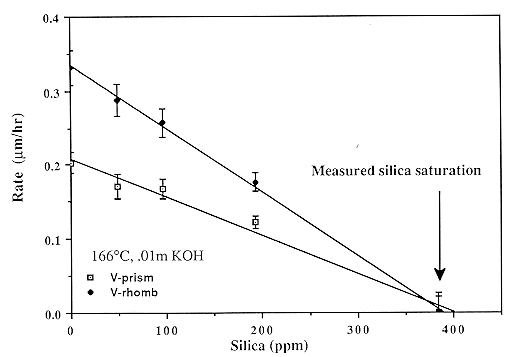29. Gratz, A. J., and P. Bird (1993a) Quartz dissolution: Negative crystal experiments and a rate law, Geochimica et Cosmochimica Acta, 57, 965-976.

Abstract. The negative crystal method is used to extend the range of measured quartz dissolution rates as a function of temperature and pOH, extent of saturation, and ionic strength. The activation energy is constant for pOH = 1 to 4, and rate decreases linearly with saturation, indicating a precipitation reaction that is first order in silicic acid. Dissolution is slowed by Ca and Mg ions; the effect of Ca is strongest on the unit rhombohedra. A simple rate law based on surface charging is proposed and shown to fit the data. Only a reaction in which the activated complex is negatively charged is consistent with rate measurements. Some constraints on fundamental kinetic parameters are inferred.

Data for all materials (single crystals and powders) can be fit by the single rate law

<Rdiss> = G A0 T S exp( -Ediss / RT ),

S = I 0.3 { 0.095 aOH-0.5 + 1}-1,

G = 1 for rough faces while G = Y exp( -D Einter / RT ) for smooth faces,

A0 = 6.64 ´ 10-3 ms s-1 K-1,

where <Rdiss> = limiting dissolution rate in m/s, S is the surface charge, aOH- is the molal hydroxide activity relative to an infinitely dilute solution at STP, I is the ionic strength, T is the temperature, D Einter is the energy of interaction (~6 kJ / mol) causing step formation on the crystal surface, Ediss is the activation energy of dissolution of ~78.6 kJ / mol, and Y is a face constant equal to 1 for the prism and 1.63 or 1.87 for the positive and negative unit rhombs, respectively. The available data in the literature suggests G = 1 to 9.5 for various other crystal directions, G = 2-4 for powders with optically estimated areas, while G = 0.1-0. 3 describes powders with BET-determined areas. This equation applies over a wide range of conditions: 25-300° C, ionic strength 0.003-0.1 m,and any pOH below the point of zero charge of quartz; a similar equation applies to growth. Dissolution mechanisms for defective crystals are discussed and may result in dissolution rate enhancements of ~2´ . The observation that these enhancements are associated with generalized etching suggests that background dissolution follows the proposed rate law, but accompanied by surface roughening and rapid removal of defect volumes.